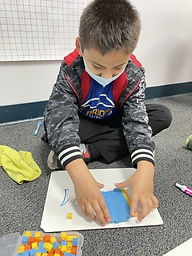Ms. Caitlyn

Target 1​

Lesson Type:

New

Number Operation

:

Integer Composition

Understand that the two digits in a double-digit number represent the number of tens and ones.

1:

Represent the composition or decomposition of two-digit numbers with an equation (13 = 10+3 or 1 ten and 3 ones).

1st

Vocabulary:

Base Ten Blocks, Tens, Ones

Activities:

• Students rolled dice to create two-digit numbers.
• Students modeled two-digit numbers using base ten blocks.
• Students wrote two-digit numbers in expanded form (i.e.: 20 + 5 = 25).Home Exploration

Guiding Questions:Absent Students:

Yilin, Naavya

Target 2

:

1:

Determine whether to round up or down to get to the nearest ten.

2:

Understand that the ones’ place is the key digit to evaluate to determine whether to round up or down.

3:

Identify which digits in the ones’ place signal to round up to the nearest ten.

4:

Identify which digits in the ones’ place signal to round down to the nearest ten.

1st

Vocabulary:

Round Up, Round Down

Activities:

• Students played a rounding game where they rounded numbers up and down a number line (1-100).Home Exploration

Guiding Questions:Target 3

:

Vocabulary:

Activities:Home Exploration